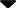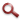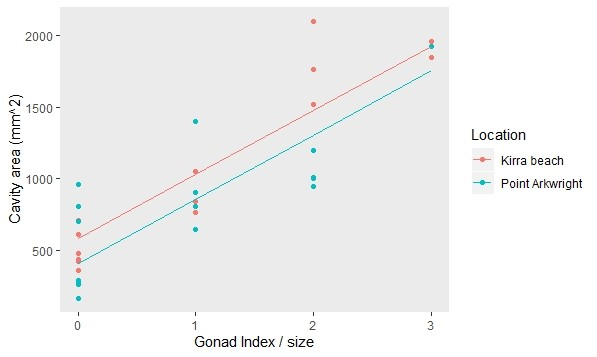Site WebYou are here:

## Student Project FiguresFigure 7 Figure 7: Scatter plot of individual Pyura praeputialis (n=30) internal cavity volume, calculated from cavity length and diameter, gonad index and sampling location. Data also suggested no effect of site on cavity area (t = 1.4208, df = 24.982, p-value = 0.1677) or external area (t = 1.7945, df = 23.169, p-value = 0.08579). Figure 7 Figure 8 Figure 9 Figure 10 Figure 11